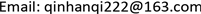1. 前言

2019年5月11日，证监会主席易会满出席中国上市公司协会2019年年会暨第二届理事会第七次会议并发表讲话中提到：近期A股面临着大幅波动的情况，股市有一定的震荡波动。资本市场是实体经济的“晴雨表”，最近一段时间以来中国股市出现了明显的波动和下滑，这是由诸多因素造成的。在我国金融体系中，由于市场形势瞬息万变，牵动着股市市场随之变化和发展。我国股票市场的波动强烈是有目共睹的，因此分析我国股市问题迫在眉睫，将如何采取最合适的手段和方法来进行有效地刻画股票市场的波动以及衡量它们的风险？

2. GARCH模型2.1. ARCH模型

ε t 2 = α 0 + α 1 ε t − 1 2 + ⋯ + α p ε t − q 2 + η t (1)

2.2. GARCH模型

GARCH与ARCH模型较为相似，是建立在ARCH模型的基础上，一种广义的表达，GARCH模型通常用于对回归或自回归模型的随机扰动项进行建模。若ARCH模型可以写成下面的形式：

h t = α 0 + α 1 ε t − 1 2 + ⋯ + α q ε t − q 2 + θ 1 h t − 1 + ⋯ + θ p h t − p (2)

h t = α 0 + ∑ i = 1 q α i ε t − i 2 + ∑ i = 1 q θ j h t − j 2 (3)

3. SV-t模型

R t = ε t e h t 2 ,     ε t ~ i .i .d t ( 0,1, ω ) (4)

h t = μ + φ ( h t − 1 − μ ) + γ t ,   γ t ~ N ( 0 , σ 2 ) (5)

4. 实证分析4.1. 数据的选择和处理

R h , t = ln h t − ln h t − 1 = ln ( h t h t − 1 ) (6)

R z , t = ln z t − ln z t − 1 = ln ( z t z t - 1 ) (7)

4.2. 数据描述性分析

4.3. 考察序列的平稳性

Statistical table of data descriptio

1%5%10%
RSH−31.53745−3.437995−2.864804−2.5685620.0000平稳
RSZ−30.17459−3.437995−2.864804−2.5685620.0000平稳

4.4. 序列的ARCH-LM效应检验

ARCH effect test result
F统计量2.559382P值0.0261
LM统计量12.70836P值0.0263

4.5. 序列的自相关以及偏自相关检验

4.6. GARCH模型实证

Comparison of test results of three model
RSH变量系数标准误差Z统计量P值
GARCH(1,1)GARCH = C(1) + C(2) * RESID(−1)2 + C(3) * GARCH(−1)
C8.65E−072.12E−074.0735800.0000
RESID(−1)20.0589690.0074847.8796220.0000
GARCH(−1)0.9328250.007136130.72140.0000
GARCH(1,2)GARCH = C(1) + C(2) * RESID(−1)2 + C(3) * GARCH(−1) +C(4) * GARCH(−2)
C1.85E−063.74E−074.9536310.0000
RESID(−1)20.0997540.0128757.7478510.0000
GARCH(−1)0.0026310.0145340.1810080.8564
GARCH(−2)0.8779850.02117841.457960.0000
GARCH(2,1)GARCH = C(1) + C(2) * RESID(−1)2 + C(3) * RESID(−2)2 + C(4) * GARCH(−1)
C9.07E−072.27E−073.9932150.0001
RESID(−1)20.0431960.0247731.7436660.0812
RESID(−2)20.0175500.0271210.6470940.5176
C9.07E−072.27E−073.9932150.0001

Parameter estimation of Shenzhen composite index return serie

C(1)−0.1550240.023034−6.7303140.0000
C(2)0.0683320.0097646.9982520.0000
C(3)−0.0285270.007489−3.8089750.0001
C(4)0.9880620.002294430.70240.0000

ARCH-M test results of Shenzhen composite index return sequenc

C(1)−0.1550240.023034−6.7303140.0000
C(2)0.0683320.0097646.9982520.0000
C(3)−0.0285270.007489−3.8089750.0001
C(4)0.9880620.002294430.70240.0000

<xref ref-type="table" rid="table">Table </xref>of estimated results for different orders of Shenzhen composite index return serie

1阶0.3519460.5532
4阶0.6704980.6126
8阶0.3936450.9243
12阶0.3715870.9733

Parameter estimation of Shenzhen composite index return serie

C(2)−0.2050700.059136−3.4677650.0005
C(3)0.1106110.0316883.4905950.0005
C(4)−0.0577660.020343−2.8396070.0045
C(5)0.9858870.005910166.80660.0000

4.7. SV模型实证

μ ∼ N ( 0 , 100 ) φ 1 ∼ B e t a ( 20 , 1.5 ) τ ∼ G a m m a ( 2.5 , 0.025 ) ω ∼ χ 2 (8)

Estimation results of parameters of MCMC algorithm in SV-T mode

μ−7.63−7.6370.47410.01734−8.561−6.676
ω6.4466.1791.5480.05754.19410.18
φ0.99770.99810.00163.117E−50.99390.9988
τ0.11140.10970.02088.754E−40.073890.1563

5. 结论与建议5.1. 主要结论

5.2. 主要建议

1) 信息披露等相关制度的健全要不断加强

2) 加强市场化建设，减少政府干预

3) 加强对投资者的教育

4) 加强证券市场法制建设